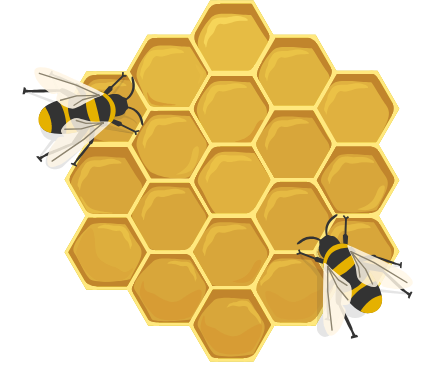## How Much Faster Together?

Suppose you and a friend can each dig a 20-foot ditch by yourselves in 3 hours. How long would it take you to dig the ditch working together, assuming you both have enough room to work without getting in each other's ways?A little reasoning tells us that the project should get finished in half the time since you're doubling your efforts by combining forces, so it should take $$1\frac12$$ hours to dig the ditch.

Now let's change things up. Suppose instead that the ditch is 40 feet long, and it takes you 8 hours to dig it by yourself. However, your friend needs more time, and it takes him 10 hours to dig the same ditch. How long would it take the two of you to dig the ditch while working together?

Since the time isn't just half—you're working at different speeds—it can be helpful to think of the rate at which you and your friend both work. For example, it takes you 8 hours to dig 1 ditch, so the rate at which you work is $$\frac{1 \text{ ditch}}{8 \text{ hours}}.$$ Meanwhile, your friend works at a rate of $$\frac{1 \text{ ditch}}{10 \text{ hours}}.$$ So together, you work at a rate of \begin{aligned}\frac{1 \text{ ditch}}{8 \text{ hours}} + \frac{1 \text{ ditch}}{10 \text{ hours}} & = \frac{5 \text{ ditches}}{40 \text{ hours}} + \frac{4 \text{ ditches}}{40 \text{ hours}} \\ \\ & = \frac{9 \text{ ditches}}{40 \text{ hours}}. \end{aligned} So how long does it take to finish the job? It's $1 \text{ ditch} \times \frac{40 \text{ hours}}{9 \text{ ditches}} = 4 \frac49 \text{ hours},$ or just under 4 hours and 27 minutes. Hooray, teamwork!

# Today's Challenge

Five worker bees work at different rates. The first bee can fill a honeycomb cell with honey by itself in one day, while the $$2^\text{nd}$$ bee takes 2 days to fill a cell by itself.

The $$3^\text{rd}$$ bee takes 3 times as long to fill a cell as the $$2^\text{nd}$$ bee, the $$4^\text{th}$$ bee takes 4 times as long as the $$3^\text{rd}$$ bee, and finally the $$5^\text{th}$$ bee takes 5 times as long as the $$4^\text{th}$$ bee.To the nearest day, how long will it take the bees to fill 10 cells with honey?

×

Problem Loading...

Note Loading...

Set Loading...